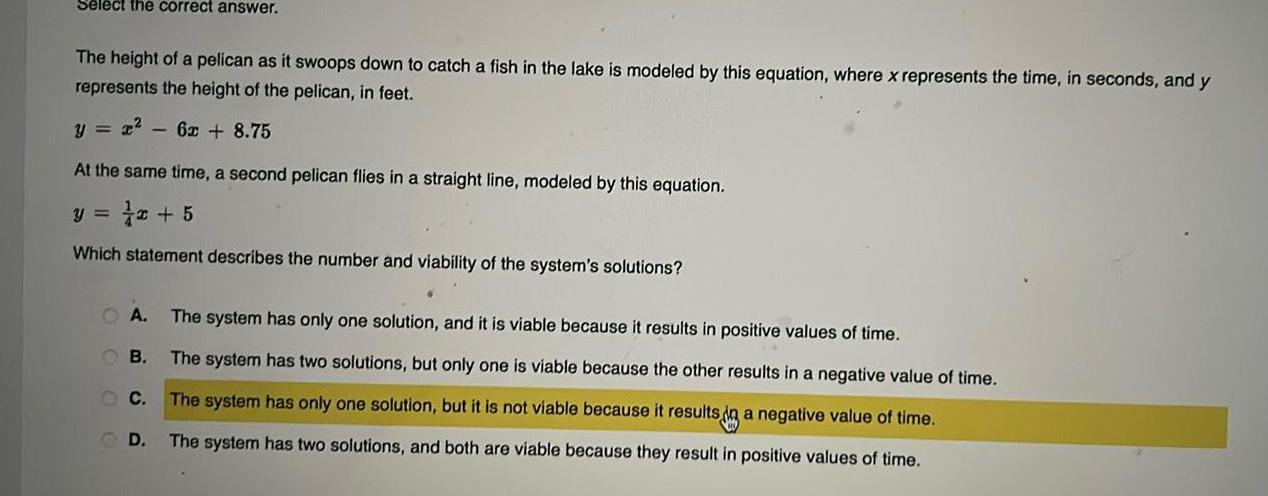Question:

# Select the correct answer The height of a pelican as it

Last updated: 11/19/2023Select the correct answer The height of a pelican as it swoops down to catch a fish in the lake is modeled by this equation where x represents the time in seconds and y represents the height of the pelican in feet y r 6x 8 75 At the same time a second pelican flies in a straight line modeled by this equation y 5 Which statement describes the number and viability of the system s solutions The system has only one solution and it is viable because it results in positive values of time The system has two solutions but only one is viable because the other results in a negative value of time C The system has only one solution but it is not viable because it results in a negative value of time D The system has two solutions and both are viable because they result in positive values of time A B